# Air Pressure at Altitude Calculator

## Calculate Altitude from Air Pressure

Pressure at Sea Level Default
Temperature Default
Air Pressure at Altitude

Altitude =

## Calculate Air Pressure at Altitude

Pressure at Sea Level Default
Temperature Default
Altitude

Air Pressure at Altitude =

Wondering what the air pressure is on Jupiter or Mars? Check out our Interplanetary Air Pressure at Altitude Calculator## Relationship Between Altitude and Pressure

The following table and graph illustrate the relationship between altitude and pressure using the default values for pressure and temperature at sea level. Using ISA standards, the defaults for pressure and temperature at sea level are 101,325 Pa and 288 K.

Altitude above Sea Level Absolute Atmospheric Pressure
feet miles meters kPa atm psia
-5000 -0.95 -1524 121.0 1.19 17.55
-4000 -0.76 -1219 116.9 1.15 16.95
-3000 -0.57 -914 112.8 1.11 16.36
-2000 -0.38 -610 108.9 1.07 15.79
-1000 -0.19 -305 105.0 1.04 15.24
-500 -0.09 -152 103.2 1.02 14.96
0 0.00 0 101.3 1.00 14.70
500 0.09 152 99.5 0.98 14.43
1000 0.19 305 97.7 0.96 14.17
1500 0.28 457 96.0 0.95 13.92
2000 0.38 610 94.2 0.93 13.66
2500 0.47 762 92.5 0.91 13.42
3000 0.57 914 90.8 0.90 13.17
3500 0.66 1067 89.1 0.88 12.93
4000 0.76 1219 87.5 0.86 12.69
4500 0.85 1372 85.9 0.85 12.46
5000 0.95 1524 84.3 0.83 12.23
6000 1.14 1829 81.2 0.80 11.78
7000 1.33 2134 78.2 0.77 11.34
8000 1.52 2438 75.3 0.74 10.92
9000 1.70 2743 72.4 0.71 10.51
10000 1.89 3048 69.7 0.69 10.11
15000 2.84 4572 57.2 0.56 8.29
20000 3.79 6096 46.6 0.46 6.75
25000 4.73 7620 37.6 0.37 5.45
30000 5.68 9144 30.1 0.30 4.36
35000 6.63 10668 23.8 0.24 3.46
40000 7.58 12192 18.8 0.19 2.72
45000 8.52 13716 14.7 0.15 2.14
50000 9.47 15240 11.6 0.11 1.68
55000 10.42 16764 9.1 0.09 1.32
60000 11.36 18288 7.2 0.07 1.04
65000 12.31 19812 5.6 0.06 0.82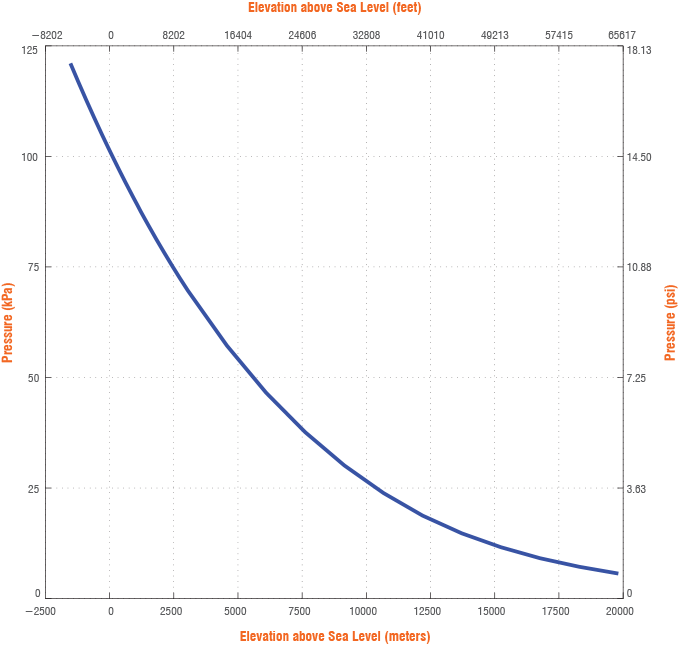Weather Conditions
Due to the fact that weather conditions affect pressure and altitude calculations, the pressure and temperature at sea level must be known. The altitude at a given air pressure can be calculated using Equation 1 for an altitude up to 11 km (36,090 feet). This equation can be arranged to also calculate the air pressure at a given altitude as shown in Equation 2.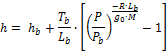(1)(2)

where,= static pressure (pressure at sea level) [Pa]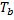= standard temperature (temperature at sea level) [K]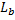= standard temperature lapse rate [K/m] = -0.0065 [K/m]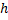= height about sea level [m]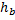= height at the bottom of atmospheric layer [m]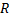= universal gas constant = 8.31432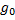= gravitational acceleration constant = 9.80665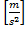= molar mass of Earth’s air = 0.0289644 [kg/mol]

Earth’s Atmosphere
Due to the fact that Earth’s atmosphere experiences different rates of heating and cooling through each of its layers, these equations help to model this through the use of the temperature lapse rate, which is the rate at which temperature changes through altitude change. Some layers, such as the stratosphere (from 11km to 20km), have a constant temperature throughout the layer. This requires different equations to determine the altitude or pressure. Equations 3 and 4 specify the calculation for altitude and pressure respectfully in this zero temperature lapse rate layer.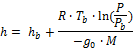(3)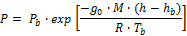(4)

For these equations,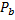, andcorrespond to the altitude, pressure, and temperature at the bottom of the stratosphere. The pressure at the bottom of the layer is determined from the user provided inputs of the pressure and temperature at sea level knowing that the altitude at the bottom of the layer is 11 km; assuming the default pressure was used at sea level, the pressure at the bottom of the stratosphere is 22,632 Pa. The temperature at the bottom of the stratosphere is determined by subtracting 71.5 K from the temperature at sea level.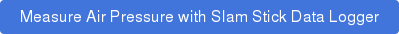page.calculators